Author: Oscar Cronquist Article last updated on March 26, 2020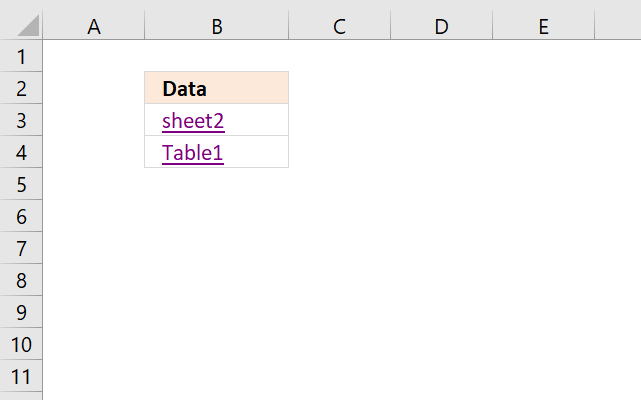The image above shows two hyperlinks, the first hyperlink lets you select a data set automatically based on a dynamic formula. The second hyperlink takes you to a specific Excel Table and automatically selecting all data as well.

The technique demonstrated in this article will be interesting for you if you often copy data sets or Excel defined tables. Adding more rows or columns to the data set makes no difference, both the first formula and the Excel defined Table formula are dynamic meaning they adapt when lists grow or shrink. This may be a time-saver for you making some actions less tedious.

Formula in cell B3:

=HYPERLINK("[Quickly select a data set or an excel defined table.xlsx]Sheet2!\$B\$2:"&ADDRESS(MATCH(CHAR(255), Sheet2!B1:B1000, 1), MATCH(CHAR(255), Sheet2!A2:CW2,1)), "sheet2")

### Explaining formula in cell B3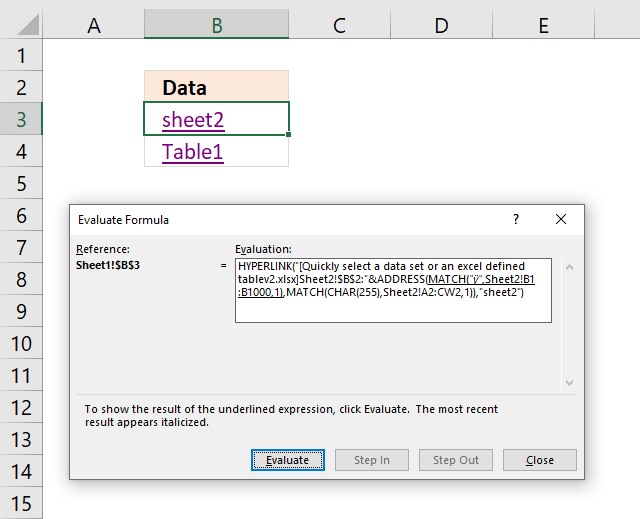I recommend that you use the "Evaluate Formula" tool built-in to Excel. Go to tab "Formulas" on the ribbon and press with left mouse button on the "Evaluate Formula" button, a dialog box appears. Press with mouse on the "Evaluate" button to see calculations step by step, see image above.

The underlined expression tells you which part of the formula that will be calculated in the next step if you press the "evaluate" button. The result is in italic making it easier for you to understand and learn how a formula works. Press with left mouse button on "Close" button to dismiss the dialog box.

#### Step 1 - Find last row in the data set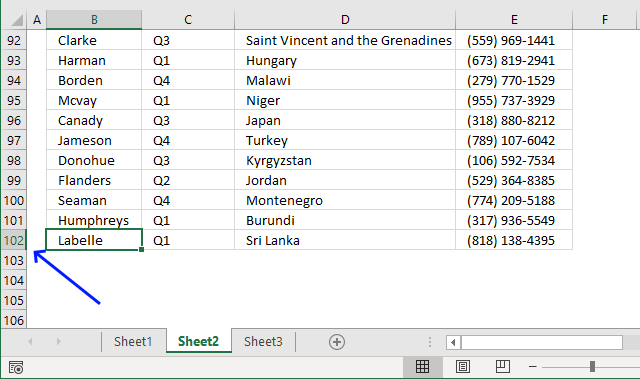The CHAR function converts a number to the corresponding character based on your computer's character set. The numbers can be from 1 to 255 and they represent a character.

MATCH(CHAR(255), Sheet2!B1:B1000, 1)

becomes

MATCH("ÿ", Sheet2!B1:B1000, 1)

The MATCH function returns a number representing the relative position of a given value in a cell range. The third argument lets you choose how the function matches the value. Number 1 lets you find the smallest value that is greater than or equal to the lookup value.

MATCH(lookup_value, lookup_array, [match_type])

The formula is looking for the last character in your computer's character set and it will most likely not be found. This will match the last value in your lookup_array. It is not required to have the column sorted in ascending order.

I recommend using multiple values concatenated like this CHAR(255)&CHAR(255) if you know that your lookup_array contains CHAR(255) .

MATCH("ÿ", Sheet2!B1:B1000, 1)

an returns 102.

Change cell range B1:B1000 if the data set has more than a thousand rows or if the data set location requires you to change the cell reference.

#### Step 2 - Find the rightmost column in the data set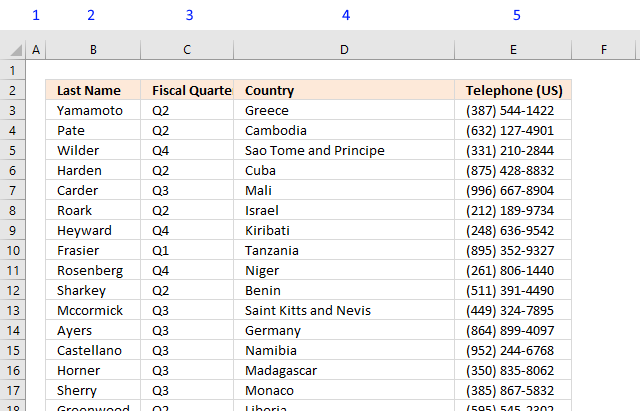The MATCH function returns the relative position of a given value in a cell range. It lets you choose how the function matches the value, use 1 in the third argument. It allows you to find the smallest value that is greater than or equal to the lookup value.

MATCH(lookup_value, lookup_array, [match_type])

We are looking for the last character in your computer's character set and it will most likely not be found. This will match the last value in your lookup_array even if it is not sorted in ascending order.

MATCH(CHAR(255), Sheet2!A2:CW2, 1)

returns 5.

This number represents the rightmost column in the data set. Change cell range A2:CW2 if the data set has more than a hundred columns.

#### Step 3 - Create cell reference

The ADDRESS function returns the address of a specific cell, based on a row and column number. We calculated the row and column numbers in steps 1 and 2.

[abs_num], [a1], and [sheet_text] are optional arguments that we don't need to use in this tutorial.

ADDRESS(MATCH(CHAR(255), Sheet2!B1:B1000, 1), MATCH(CHAR(255), Sheet2!A2:CW2, 1))

becomes

returns cell reference \$E\$102.

#### Step 4 - Add file and sheet name

The HYPERLINK function requires the workbook name and worksheet name to operate properly. The ampersand character lets you concatenate two strings, in this case, the workbook name, the worksheet name, and the cell reference.

The workbook name must have a beginning and ending bracket and ther worksheet name must end with an exclamation mark.

"[Quickly select a data set or an excel defined table.xlsx]Sheet2!\$B\$2:"&ADDRESS(MATCH(CHAR(255), Sheet2!B1:B1000, 1), MATCH(CHAR(255), Sheet2!A2:CW2, 1))

becomes

"[Quickly select a data set or an excel defined table.xlsx]Sheet2!\$B\$2:"&\$E\$102)

becomes

[Quickly select a data set or an excel defined table.xlsx]Sheet2!\$B\$2:\$E\$102

#### Step 5 - Create the hyperlink

The HYPERLINK function lets you build a hyperlink in your worksheet using a formula. A formula can be made dynamic so if your data set changes the formula changes as well without any user interaction.

HYPERLINK("[Quickly select a data set or an excel defined table.xlsx]Sheet2!\$B\$2:"&ADDRESS(MATCH(CHAR(255), Sheet2!B1:B1000, 1), MATCH(CHAR(255), Sheet2!A2:CW2,1)), "sheet2")

becomes

HYPERLINK([Quickly select a data set or an excel defined table.xlsx]Sheet2!\$B\$2:\$E\$102, "sheet2")Formula in cell B4:

=HYPERLINK("[Quickly select a data set or an excel defined table.xlsx]Sheet2!Table1[#All]", "Table1")

### Select Excel defined Table using a hyperlink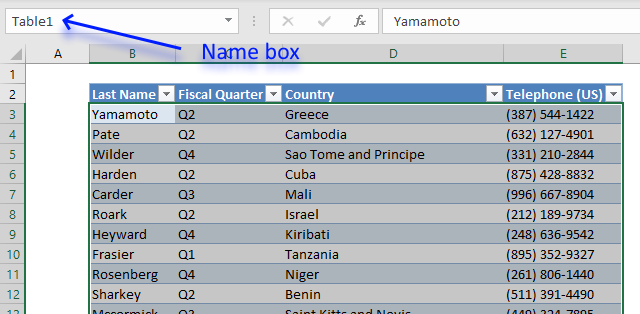The Excel Table is great a built-in tool that has many advantages. This tutorial demonstrates how Excel uses cell references to Excel Tables. They are called "structured references" and work differently than regular cell references.

A structure reference begins with the Excel Table name, press with left mouse button on any cell in the Excel Table. Press with mouse on tab "Table Design" on the ribbon, the tab appears if you have a cell in the Excel Table selected. You can find the Table name on the ribbon, it also allows you to change the Table name if you want to.

The name box shown in the image above lets you try different "structured references" and see what they do. The table below shows different "structured references", type them in the name box and check out what Excel selects.

For example, you will select data in the Excel Table if you only reference the Excel Table name. Structured references allow you to also use square brackets as well to further specify data you want to reference in the Excel Table.

 Structured reference Description Table1[#All] Selects everything, headers, and data. Table1 Selects data. Table1[[#All],[Country]] Selects data and column header "Country". Table1[Country] Selects data in column Country

### Get the Excel fileQuickly-select-a-data-set-or-an-excel-defined-tablev2.xlsx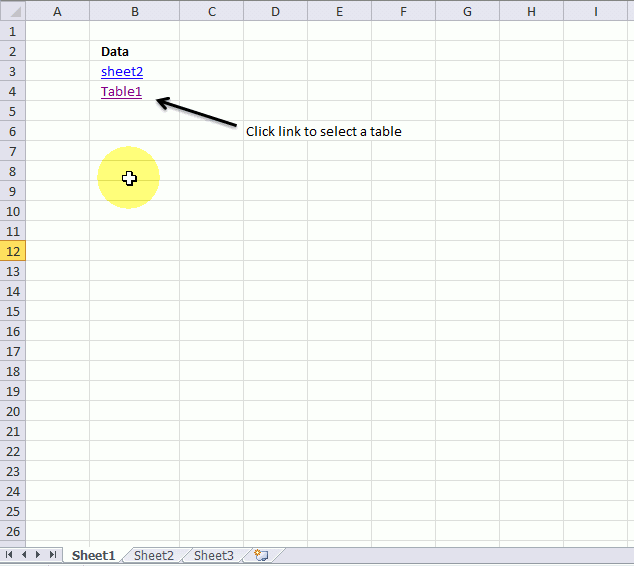The animated gif shows two hyperlinks, the first one selects a data set, the second one selects an excel defined table.